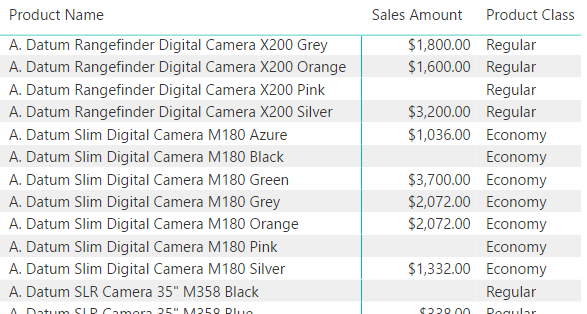The DAX language is growing thanks to the monthly updates of Power BI, which gradually introduce new features later made available also in Analysis Services and Power Pivot. The July 2017 release of Power BI Desktop includes a new tool function called SELECTEDVALUE. This function does not introduce anything new – it just simplifies the syntax required in common coding patterns, as you will see in a few of the possible uses presented after the description of the SELECTEDVALUE syntax.

## SELECTEDVALUE Syntax

The function SELECTEDVALUE returns the value of the column reference passed as first argument if it is the only value available in the filter context, otherwise it returns blank or the default value passed as second argument. Here are a few examples of possible syntax.

```SELECTEDVALUE ( Table[column] )
SELECTEDVALUE ( Table[column], "default value" )
SELECTEDVALUE ( Table[column], 0 )
```

Internally, SELECTEDVALUE is just syntax sugar generating the following corresponding syntaxes:

```IF ( HASONEVALUE ( Table[column] ), VALUES ( Table[column] ) )
IF ( HASONEVALUE ( Table[column] ), VALUES ( Table[column] ), "default value" )
IF ( HASONEVALUE ( Table[column] ), VALUES ( Table[column] ), 0 )
```

You should use SELECTEDVALUE in all those cases when you need to read a single value selected in the filter context, obtaining blank or another default value in all other cases.

It is important to remember that:

• The SELECTEDVALUE and VALUES functions read the current filter context, not the row context;
• When you have a row context, just use a column reference (within RELATED in case it is in a lookup table);
• If the filter context returns zero rows for the referenced column, then SELECTEDVALUE returns the second argument – do not assume that the second argument is returned only when two or more values are selected;
• If you use a version of DAX that does not have SELECTEDVALUE, you can use the same pattern as that described in this article, replacing SELECTEDVALUE with the corresponding syntax using HASONEVALUE / VALUES.

## Sample Uses

The Power BI file you can download contains all the examples described in this section.

## Retrieving a column from the same table

You can retrieve the attribute of an entity (e.g. the current class of a product) displayed in a matrix, making sure you are not going to duplicate the rows in case there are more attribute values available for that same selection.

```Product Class := SELECTEDVALUE ( 'Product'[Class] )
```This measure is useful when you navigate using a Matrix or a PivotTable. However, the measure displays data even for products that have no sales. This does not happen when you use a Table visual in Power BI.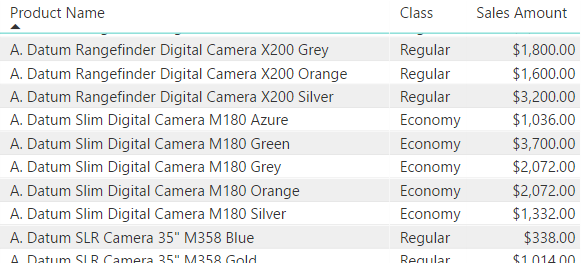If you want to show the class of a product only when there is a measure available, you should add a condition as in the two following examples:

```Product Class v1 :=
IF (
NOT ISBLANK ( [Sales Amount] ),
SELECTEDVALUE ( 'Product'[Class] )
)

Product Class v2 :=
IF (
NOT ISEMPTY ( Sales ),
SELECTEDVALUE ( 'Product'[Class] )
)
```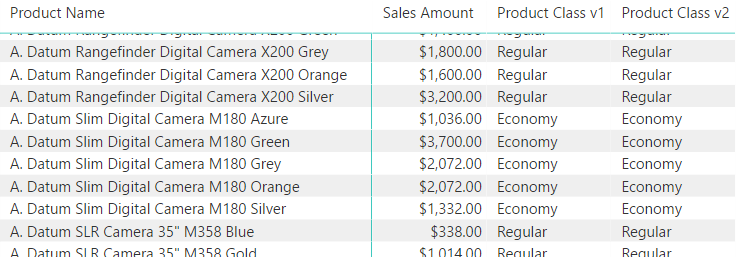## Retrieving a column from a lookup table

If you want to retrieve the corresponding attribute in a lookup table, you probably need to modify the propagation of the filter context through relationships. When you have a row context you would use a single RELATED function to retrieve the corresponding row context in a lookup table, regardless of the number of relationships to travel. For example, consider the case of a snowflake dimension featuring the Product, Product Subcategory, and Product Category tables. If you want to get the current category of a product in a Matrix or in a PivotTable, you need the following code:

```Product Category :=
CALCULATE (
SELECTEDVALUE ( 'Product Category'[Category] ),
CROSSFILTER (
'Product'[ProductSubcategoryKey],
'Product Subcategory'[ProductSubcategoryKey],
Both
),
CROSSFILTER (
'Product Subcategory'[ProductCategoryKey],
'Product Category'[ProductCategoryKey],
Both
)
)
```

In this case, a simpler syntax could be the one provided by the expanded table:

```Product Category exp.table :=
CALCULATE (
SELECTEDVALUE ( 'Product Category'[Category] ),
'Product'
)
```

However, remember that the expanded table might have undesired side effects in complex data models, so the CROSSFILTER solution should be preferred. Both measures return the same result.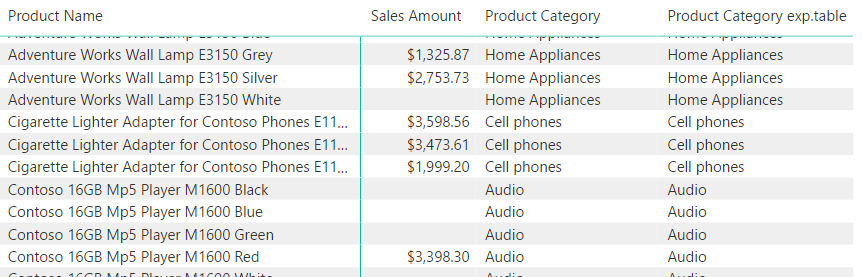## Using a numeric column in a calculation

The SELECTEDVALUE function simplifies the syntax required when you use a numeric column of an entity as a parameter in a calculation. For example, the following measure calculates the quantity dividing the existing Sales Amount measure by the Unit Price value of the selected product. In case more products are selected, the blank result of SELECTEDVALUE automatically propagates into the result of Calc Quantity.

```Calc Quantity :=
DIVIDE (
[Sales Amount],
SELECTEDVALUE ( 'Product'[Unit Price] )
)
```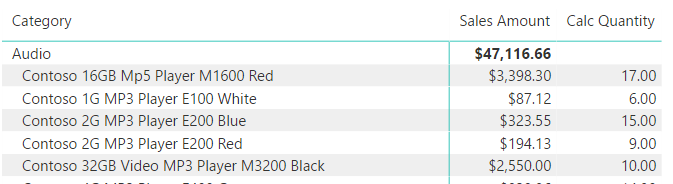## Parameter table pattern

If you consider the parameter table pattern, you can implement a measure using a parameter by using SELECTEDVALUE writing this:

```Sales by Scale :=
DIVIDE (
[Sales Amount],
SELECTEDVALUE ( 'Scale'[Scale], 1 )
)
```

```Sales by Scale :=
DIVIDE (
[Sales Amount],
IF ( HASONEVALUE ( Scale[Scale] ), VALUES ( Scale[Scale] ), 1 )
)
```

The result is the same, you just simplify your code.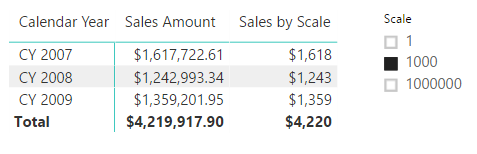If you want to avoid a multiple selection, you could use the ERROR function, so the visual displays a proper error message (even if the reference to MdxScript seems like nonsense in a DAX client such as Power BI Desktop).

```Sales by Scale Checked :=
DIVIDE (
[Sales Amount],
SELECTEDVALUE ( 'Scale'[Scale], ERROR ( "Single selection required" ) )
)
```## Conclusion

The SELECTEDVALUE function simplifies the syntax of a common pattern involving two functions (HASONEVALUE and VALUES) to retrieve a value from the filter context. Just remember that this technique is not required when you have a row context, because you can simply use a column reference and the RELATED function when a lookup table is involved.